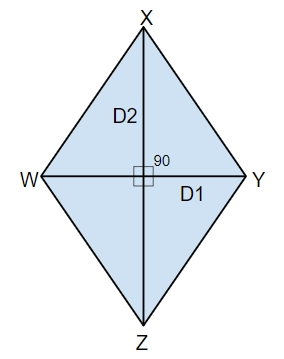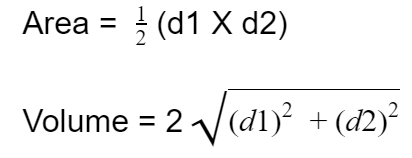# Program to calculate area and perimeter of a rhombus whose diagonals are givenWhat is rhombus in C++?

## What is rhombus?

In geometry, rhombus is a quadrilateral with four sides of same length. Rhombus resembles with the shape diamond. If the diagonals of rhombus meet at right angle than it becomes square.

Properties of rhombus are −

• equal sides
• Opposite sides are parallel and opposite angles are equal making it parallelogram
• diagonals bisects at right angle

Given below is the figure of rhombus## Problem

Given with the diagonals, let’s say, d1 and d2 the task is to find the area and perimeter of a rhombus where area is the space occupied by the shape and perimeter is the space that its boundaries will cover

To calculate area and perimeter of a cuboid there is a formula −## Example

Input-: d1=6 and d2=12
Output-: The perimeter of rhombus with given diagonals are :26
The area of rhombus with given diagonals are :36

## ALGORITHM

Start
Step 1 -> declare function to calculate perimeter of rhombus
int perimeter(int d1, int d2)
Declare variable long long int perimeter
Set perimeter = 2 * sqrt(pow(d1, 2) + pow(d2, 2))
Print perimeter
Step 2 -> Declare function to calculate area of rhombus
int area(int d1, int d2)
Declare long long int area
Set area = (d1 * d2) / 2
Print area
Step 3 -> In main()
Declare variable int d1 = 6, d2 = 12
Call perimeter(d1, d2)
Call area(d1, d2)
Stop

## Example

#include <iostream>
#include <math.h>
using namespace std;
// program to calculate perimeter of rhombus
int perimeter(int d1, int d2){
long long int perimeter;
perimeter = 2 * sqrt(pow(d1, 2) + pow(d2, 2));
cout<< "The perimeter of rhombus with given diagonals are :"<<perimeter;
}
//program to calculate area of rhombus
int area(int d1, int d2){
long long int area;
area = (d1 * d2) / 2;
cout<<"The area of rhombus with given diagonals are :"<< area;
}
int main(){
int d1 = 6, d2 = 12;
perimeter(d1, d2);
area(d1, d2);
return 0;
}

## Output

The perimeter of rhombus with given diagonals are :26
The area of rhombus with given diagonals are :36

Updated on: 20-Sep-2019

102 Views